# HC Verma Solutions Class 12 Chapter 18 Electromagnetic Waves

HC Verma Solutions Class 12 Chapter 18 Electromagnetic Waves are available here to help students find the right answers to questions related to Coulomb’s law, magnetic field, electric field and the velocity of the particle, etc. With detailed analysis, we have provided a thorough solution with a contemporary approach to help students further understand all the problems given in this chapter. They will be able to understand the topics given in electromagnetic waves in a better way.

As for the problems students will have to deal with questions like:

• Finding the intensity of the wave when the electric fields and magnetic fields are given.
• Finding the average energy density when the magnetic field is given.
• Finding the dimensional formula of quantities like the magnetic field at zero position and we have questions related to finding the free current at the zero position.

The HC Verma solutions that we are offering have been prepared by experts and will seemingly provide students with the right skillset to solve even the most complex problems. The solution also contains exercises which are arranged in a systematic manner in order to give you an amazing learning experience while studying the chapter.

## Fundamental Topics Related To Electromagnetic Waves

Some of the important topics discussed in this chapter are;

• Maxwell’s Displacement Current
• Continuity of Electric Current
• Maxwell’s Equations and Plane Electromagnetic Waves
• Energy density and Intensity
• Momentum
• Electromagnetic Spectrum and Radiation in the Atmosphere

## Class 12 Important Questions In Chapter 18

1. State whether an electromagnetic wave can be deflected by an electric field or a magnetic field?

2. If we take a metal rod and place it along the axis of a solenoid with high-frequency alternating current the metal rod starts heating up. Explain why this happens.

3. What produces electromagnetic waves?

(a) an accelerating charge (b) a charge that is static (c) moving charge (d) chargeless particles

4. When a free electron is placed in the path of a plane electromagnetic wave in which direction will the electron move?

(a) in the direction of propagation of the wave (b) in a plane containing the magnetic field and the direction of propagation (c) along the magnetic field (d) across the electric field

5. For which quantity will the speed of electromagnetic waves be the same?

(a) for all frequencies (b) for all intensities (c)  for all wavelengths (d) in all media

## HC Verma Solutions Vol 2 Electromagnetic Waves Chapter 18

Question 1: Show that the dimensions of the displacement current Єo $\frac{d \phi_E}{dt}$ are that of an electric current.

Solution:

The displacement current Id = Єo $\frac{d \phi_E}{dt}$  …(1)

Where φE is the electric flux produced by the time varying electric field.

According to Gauss’s law, the electric flux φE through a surface:

φE = qenclosedo

(1)=>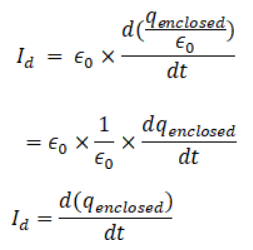Now,

Dimension of Id = [TA]/[T] = [A]

As [A] is the dimension of electric current which are

Since dimension of displacement current has same dimension as that of electric current.

So, dimension of displacement current = [A]

Question 2: A point charge is moving along a straight line with a constant velocity v. Consider a small area A perpendicular to the direction of motion of the charge figure. Calculate the displacement current through the area when its distance from the charge is x. The value of x is not large so that the electric field at any instant is essentially given by Coulomb’s law.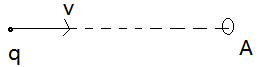Solution:

The electric field produced by the charge when it is at a distance x from the surface is given by Coulomb’s law is E i.e.

E = q/(4πЄox2)

And E produces an electric flux through the area whose magnitude is given by Gauss’s law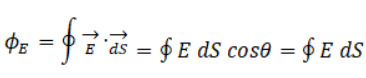As, electric field lines are directed along the normal to the area vector of the surface.

So, φE = EA = qA/(4πЄox2)

The displacement current through the area: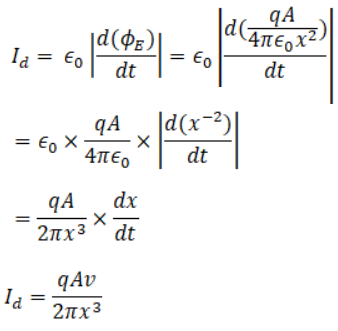Where dx/dt = v, as “x” is the distance of the charge from the area at different intervals of time.

Question 3: A parallel-plate capacitor having plate-area A and plate separation d is joined to a battery of emf ϵ and internal resistance R at t = 0.

Consider a plane surface of area A/2, parallel to the plates and

situated symmetrically between them. Find the displacement current

through this surface as a function of time.

Solution: The displacement current Id is generated due to the fact that the charge on capacitor plates is changing with time.

Id = Єo |d/dt (φE)|

If the charge on the capacitor plate is Q and the area of the plate is “A”, then

∮E. ds = Q/Єo

If dS is a small area element on the plate.

∮E.ds = ∮Eds cos 0o = EA

=> EA = Q/Єo

or E = Q/AЄo, which is the electric field between the plates.

This electric field produces and electric flux through the plane surface.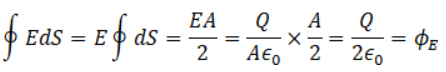If the capacitance of the capacitor is C, then the charge Q at time t is

Q = εC(1 – e-t/RC)

Now,

The displacement current as a function of time, Id: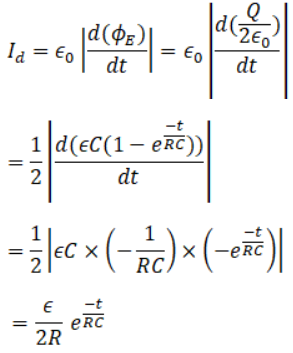Question 4: Consider the situation of the previous problem. Define displacement resistance Rd = V/id of the space between the plates where V is the potential difference between the plates and id is the displacement current. Show that Rd varies with time as Rd = R (et/τ – 1)

Solution:

Displacement current = Id = Єo |d/dt (φE)|

Electric flux, Using Gauss’s law:

φE = Q/Єo

Where, Q = charge enclosed

And Q = CV = CVo e(-t/RC)

[As the capacitor is charging, the charge will be a function of time]

Now, the flux is:

φE = [CVo e(-t/RC)]/ Єo

E = q/(4πЄox2)

Now, let us find the displacement current: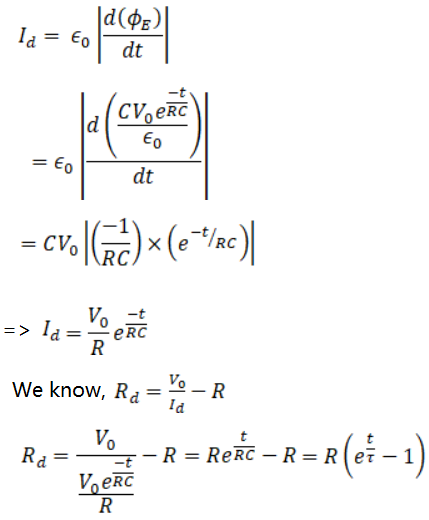Here τ is the time constant, thus Rd varies with time.

Question 5: Using B = μ0 H find the ratio E0/H0 for a plane electromagnetic wave propagating through vacuum. Show that it has the dimensions of electric resistance. This ratio is a universal constant called the impedance of free space.

Solution:

B0 = E0/C ….(1)

Also, for free space given relation can be written as, B0 = μ0 Ho …(2)

From (1) and (2), we have

μ0 Ho = E0 /C

=>Eo/Ho = μ0 C …(3)

Dimension of C = [L T-1]

Find the dimension of μ0:

According to scalar form of Biot Savart law:

B = μ0/4π x iL/R2

Or μ0 = [4πR2B] / iL

Dimensions of μ0 = [MT-2A-1][L2]/[L][A] = [MLT-2A-2]

(3)=> Dimensions of Eo/Ho = [MLT-2A-2] [L T-1] = [ML2T-3A-2]

Therefore, Dimensions of Eo/Ho has the same dimensions as that of electrical resistance i.e. [R].

Question 6: The sunlight reaching the earth has maximum electric field of 810 V m–1. What is the maximum magnetic field in this light?

Solution:

We know, maximum magnetic field = B0 = E0/C

Where, C is the speed of light in vacuum and B0 is the amplitude of maximum magnetic field.

B0 = E0/C = 810/[3×108] = 2.7 x 10-6 T = 2.7 μ T

Question 7: The magnetic field in a plane electromagnetic wave is given by

B = (200 μT) sin [(4.0 × 1015 s–1) (t–x/c)].

Find the maximum electric field and the average energy density corresponding to the electric field.

Solution:

Given: B = (200 μT) sin [(4.0 × 1015 s–1) (t–x/c)]

We know, relation between magnetic field and electric field : B0 = E0/C

The electric field intensity: E0 = B0 C

=> E0 = (3×108) x 200 = 6 x 104 N/C

The energy density associated with an electric field:

Ud = (1/2) Єo Eo2

Ud = (1/2) x (8.85 x10-12) x (6×104)2

= 159.3 x 10-4 Jm-3

=> Ud = 1.593 x 10-2 Jm-3

Question 8: A laser beam has intensity 2.5 × 1014 W m–2. Find the amplitudes of electric and magnetic fields in the beam.

Solution:

The amplitude of the electric field is related to the intensity of the wave by the relation:

I = (1/2) Єo Eo2 C

or Eo2 = 2I/ ЄoC

= [2×2.5×1014]/[3×108x8.85×10-12]

Or Eo = 4.339 x 108 NC-1

=>Electric field amplitude = Eo = 4.339 x 108 NC-1

The relation between amplitudes of electric and magnetic fields is given as:

Bo = Eo/C

So, magnetic field amplitude = Bo = [4.339×108]/[3×108] = 1.43 T

Question 9: The intensity of the sunlight reaching Earth is 1380 W m−2. Assume this light to be a plane, monochromatic wave. Find the amplitudes of electric and magnetic fields in this wave.

Solution:

The relation between amplitude of the electric field and the intensity of the wave is

I = (1/2) Єo Eo2 C

or Eo2 = 2I/ ЄoC

= [2×1380]/[3×108x8.85×10-12]

Or Eo = 1.02 x 103 NC-1

=>Electric field amplitude = Eo = 1.02 x 103 NC-1

The relation between amplitudes of electric and magnetic fields is given as:

Bo = Eo/C

Value of Magnetic field amplitude = Bo = [1.02×103]/[3×108] = 3.4 x 10-6 T = 3.4 μT.